### 15.5.2.2 First-order controllable systems

The approach developed for the nonholonomic integrator generalizes to systems of the form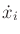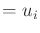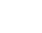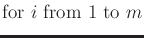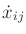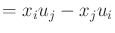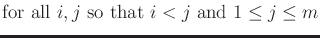(15.155)

and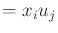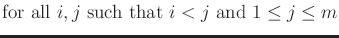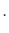(15.156)

Brockett showed in  that for such first-order controllable systems, the optimal action trajectory is obtained by applying a sum of sinusoids with integrally related frequencies for each of the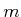action variables. Ifis even, then the trajectory for each variable is a sum of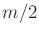sinusoids at frequencies,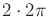,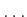,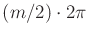. Ifis odd, there are instead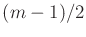sinusoids; the sequence of frequencies remains the same. Supposeis even (the odd case is similar). Each action is selected as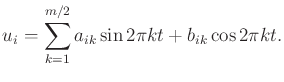(15.157)

The other state variables evolve as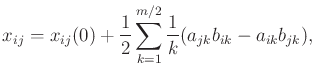(15.158)

which provides a constraint similar to (15.153). The periodic behavior of these action trajectories causes the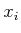variables to return to their original values while steering the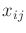to their desired values. In a sense this is a vector-based generalization in which the scalar case was the nonholonomic integrator.

Once again, a two-phase steering approach is obtained:

1. Apply any action trajectory that brings everyto its goal value. The evolution of thestates is ignored in this stage.
2. Apply sinusoids of integrally related frequencies to the action variables. Choose each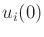so thatreaches its goal value. In this stage, thevariables are ignored because they will return to their values obtained in the first stage.

This method has been generalized even further to second-order controllable systems:(15.159)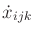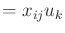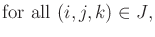in which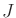is the set of all unique triples formed from distinct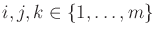and removing unnecessary permutations due to the Jacobi identity for Lie brackets. For this problem, a three-phase steering method can be developed by using ideas similar to the first-order controllable case. The first phase determines, the second handles, and the third resolves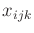. See [727,846] for more details.

Steven M LaValle 2020-08-14